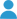By Rusty

# Introduction

This calculator finds the buoyancy of an object based on its displacement. It also uses the object weight or density to find the net buoyancy.

# Calculator

(success) Enter volume followed by unit. (e.g. "0.1 m^3" or "20 in^3")
(success) Enter weight or density followed by unit. (e.g. "2.1 kg" or "12 lb/ft^3")

Net Buoyancy
Buoyancy
Weight
Average Density

# Densities of Common Materials

Material Density (click to use above)